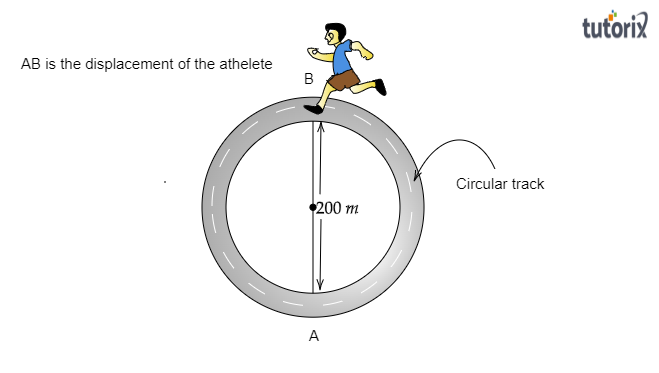# An athlete completes one round of a circular track of diameter 200 m in 40 s. What will be the distance covered and the displacement at the end of 2 minutes 20 s?

Given:

An athlete completes one round of a circular track of diameter $200\ m$ in $40\ s$.

To do:

To find the distance covered and the displacement at the end of 2 minutes 20 seconds

Solution:

As given, the diameter of the circular track $d=200\ m$

Therefore, the radius of the circular track $r=\frac{200\ m}{2}=100\ m$

Therefore, the circumference of the circular track $=2\pi r$

$=2\times \pi\times 100=200\pi\ m$

The circumference of this circular track is equal to the distance covered to complete a round.

Therefore, the distance covered to complete a round$=200\pi\ m$

It is also given that the athlete completes one round of a circular track in $40\ s$.

Therefore, speed of the athlete $=\frac{distance}{time}$

$=\frac{200\pi}{40}=5\pi\ m/s$

Now we have to find out the distance covered and the displacement at the end of 2 minutes 20 s.

Distance covered at the end of 2 minutes 20 s

Here, time taken $=$ 2 minutes 20 s$=2\times60+20=140\ s$

Distance traveled in the given time$=speed\times time$

$=5\pi \times 140$

$=700\ pi\ m$

$=700\times \frac{22}{7}\ m$

$=2200\ m$

Therefore, the distance covered by the athlete at the end of 2 minutes 20 s is $2200\ m$.

Displacement at the end of 2 minutes 20 s

Displacement is the distance between the final point and the initial point. Let's find the position of the athlete at the end of 2 minutes 20 s.

We know that $200\pi$ is equal to the distance covered to complete one round.

Therefore, the number of rounds to cover $700\pi$ distance$=\frac{700\pi}{200\pi}=3.5$

Therefore, the athlete completes three complete and a half rounds in 2 minutes 20 s. At the end of 2 min and 20 sec, he would be exactly diametrically opposite to the starting point.Therefore, the displacement of the athlete is the diameter of the circular track$=200\ m$.